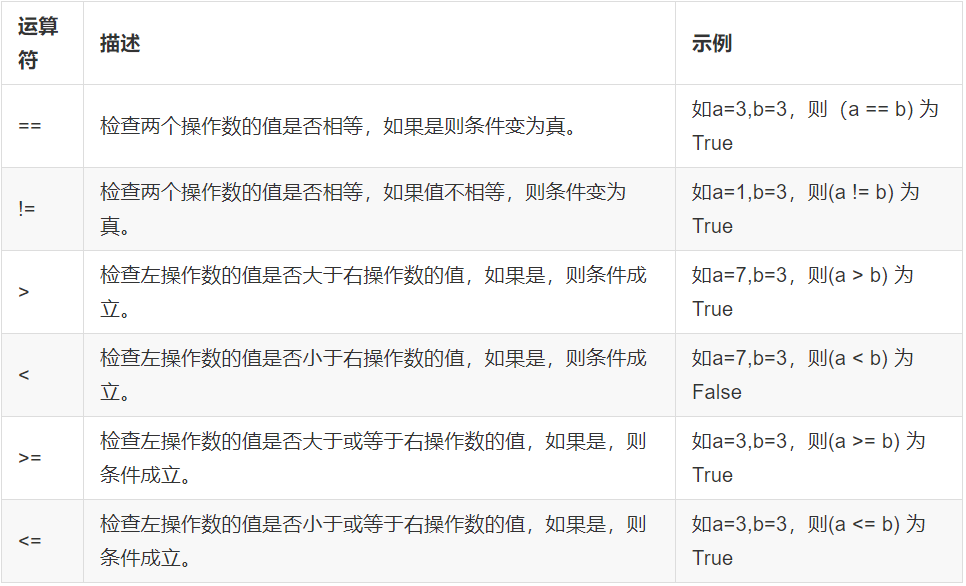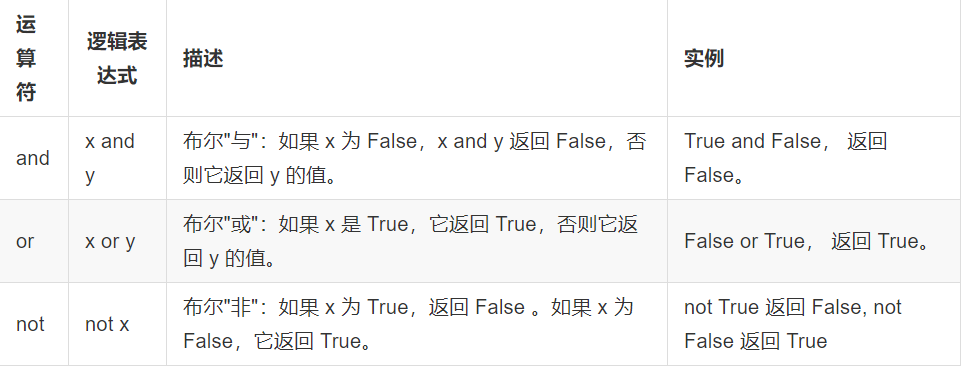# Python语法-判断和循环语句（explain）,Phython,详解

### 一.if判断语句基本格式介绍

• if语句是用来进行判断的，其使用格式如下：
``````if 要判断的条件:
条件成立时，要做的事情
``````
• Demo
``````age = 30

print("------if判断开始------")

if age >= 18:
print("我已经成年了")

print("------if判断结束------")
``````
``````------if判断开始------

------if判断结束------
``````
• 就是当满足一定条件时才会执行代码块语句，否则就不执行代码块语句。
• 注意：代码的缩进为一个tab键，或者4个空格

### 二.比较(即关系，条件)运算符

• (1)python中的比较运算符如下表``````num1 = 15
num2 = 20
# == 等于：表示左右两个操作数是否相等，如果相等则整个表达式的值为 True；不相等则为False
print(num1 == num2)

# != 不等于
print(num1 != num2)
``````
``````False
True
``````

• (2)逻辑运算符``````# and : 左右表达式都为True，整个表达式结果才为 True
if (1 == 1) and (10 > 3):
print("条件成立！")

# or : 左右表达式有一个为True，整个表达式结果就为 True
if (1 == 2) or (10 > 3):
print("条件成立！")

# not：将右边表达式的逻辑结果取反，Ture变为False，False变为True
if not (1 == 2):
print("条件成立！")
``````
``````条件成立！

``````

### 三.if-else

• if-else的使用格式
``````if 条件:
满足条件时要做的事情1
满足条件时要做的事情2
满足条件时要做的事情3
...(省略)...
else:
不满足条件时要做的事情1
不满足条件时要做的事情2
不满足条件时要做的事情3
...(省略)...
``````
• Demo
``````chePiao = 1  # 用1代表有车票，0代表没有车票
if chePiao == 1:
print("有车票，可以上火车")
print("终于可以见到Ta了，美滋滋~~~")
else:
print("没有车票，不能上车")
print("亲爱的，那就下次见了"）
``````
• 结果1：有车票的情况
``````有车票，可以上火车

``````
• 结果2：没有车票的情况
``````没有车票，不能上车

``````

### 四.if…elif…else…语句格式

• elif的功能
``````#elif的使用格式如下:
if xxx1:
事情1
elif xxx2:
事情2
elif xxx3:
事情3
``````
• 说明:
• 当xxx1满足时，执行事情1，然后整个if结束
• 当xxx1不满足时，那么判断xxx2，如果xxx2满足，则执行事情2，然后整个if结束
• 当xxx1不满足时，xxx2也不满足，如果xxx3满足，则执行事情3，然后整个if结束

• demo
``````score = 77

if score >= 90 and score <= 100:
print('本次考试，等级为A')
elif score >= 80 and score < 90:
print('本次考试，等级为B')
elif score >= 70 and score < 80:
print('本次考试，等级为C')
elif score >= 60 and score < 70:
print('本次考试，等级为D')
elif score >= 0 and score < 60:
print('本次考试，等级为E')
``````
``````本次考试，等级为C
``````

• 注意点
• 可以和else一起使用
``````if 性别为男性:
输出男性的体重
...
elif 性别为女性:
输出女性的体重
...
else:
第三种性别的体重
...
``````
• elif必须和if一起使用，否则出错
• else 一般用在最后，即所有条件都不满足时使用

• if 实现三目运算操作
• a if a > b else b
• 如果 a > b的条件成立,三目运算的结果是a,否则就是b
``````# 求a和b两个数字中的较大值.
a = 10
b = 20
# 使用三目运算符求较大值
max = a if a > b else b
print("较大值为:%d" % max)
``````
``````较大值为:20
``````

### 五.if嵌套

• if嵌套的格式
``````if 条件1:

满足条件1 做的事情1
满足条件1 做的事情2

if 条件2:
满足条件2 做的事情1
满足条件2 做的事情2
``````
``````说明

``````
• demo
``````chePiao = 1     # 用1代表有车票，0代表没有车票
daoLenght = 9     # 刀子的长度，单位为cm

if chePiao == 1:
print("有车票，可以进站")
if daoLenght < 10:
print("通过安检")
print("终于可以见到Ta了，美滋滋~~~")
else:
print("没有通过安检")
print("刀子的长度超过规定，等待警察处理...")
else:
print("没有车票，不能进站")
print("亲爱的，那就下次见了")
``````
``````有车票，可以进站

``````
• chePiao = 0;daoLenght = 9
``````没有车票，不能进站

``````

### 六.While循环语句

• while循环的格式
``````while 条件:
条件满足时，做的事情1
条件满足时，做的事情2
条件满足时，做的事情3
...(省略)...
``````
• 软件开发中循环的使用场景
• 跟媳妇承认错误，说一万遍"媳妇儿，我错了"
``````print("媳妇儿，我错了")
print("媳妇儿，我错了")
print("媳妇儿，我错了")
...(还有99997遍)...
``````
• 使用循环语句一句话搞定
``````i = 0
while i < 10000:
print("媳妇儿，我错了")
i += 1
``````
• 循环不是必须要使用的，但是为了 ``` 提高代码的重复使用率 ``` ，所以有经验的开发者都会采用循环

``````#encoding=utf-8

i = 1
sum = 0
while i <= 100:
sum = sum + i
i += 1

print("1~100的累积和为:%d" % sum)
``````

``````#encoding=utf-8

i = 1
sum = 0
while i <= 100:
if i % 2 == 0:
sum = sum + i
i+=1

print("1~100的累积和为:%d" % sum)
``````
``````1~100的累积和为:2550
``````

### 七.while循环嵌套

``````while 条件1:

条件1满足时，做的事情1
条件1满足时，做的事情2
条件1满足时，做的事情3
...(省略)...

while 条件2:
条件2满足时，做的事情1
条件2满足时，做的事情2
条件2满足时，做的事情3
...(省略)...
``````

• 要求：打印如下图形：
`````` *
* *
* * *
* * * *
* * * * *
``````
• 参考代码
``````i = 1
while i <= 5:
j = 1
while j <= i:
print("*", end=" ")
j += 1
print()

i += 1
``````

### 八.for循环

• 像while循环一样，for可以完成循环的功能。
• 在Python中 for循环可以遍历任何序列的项目，如一个列表或者一个字符串等。
• for循环的格式
``````for 临时变量 in 列表或者字符串等可迭代对象:
循环满足条件时执行的代码
``````
``````name = 'hello'

for x in name:
print(x)
if x == 'l':
print("Hello world!")
``````
``````h
e
l
Hello world!
l
Hello world!
o
``````

### 九.break和continue

• break
• ``` <1> for循环 ```
``````name = 'hello'

for x in name:
print('----')
print(x)
else:
print("==for循环过程中，如果没有执行break退出，则执行本语句==")
``````
``````----
h
----
e
----
l
----
l
----
o
==for循环过程中，如果没有执行break退出，则执行本语句==
``````
• 带有break的循环示例如下:
``````name = 'hello'

for x in name:
print('----')
if x == 'e':
break
print(x)
else:
print("==for循环过程中，如果没有执行break退出，则执行本语句==")
``````
``````----
h
----
``````
• ``` <2> while循环 ```
``````i = 0

while i<5:
i = i+1
print('----')
print(i)
else:
print("==while循环过程中，如果没有执行break退出，则执行本语句==")
``````
``````----
1
----
2
----
3
----
4
----
5
==while循环过程中，如果没有执行break退出，则执行本语句==
``````
• 带有break的循环示例如下:
``````i = 0

while i<5:
i = i+1
print('----')
if i==3:
break
print(i)
else:
print("==while循环过程中，如果没有执行break退出，则执行本语句==")
``````
``````----
1
----
2
----
``````
• 小结:break的作用：立刻结束break所在的循环

• continue
• ``` <1> for循环 ```
``````name = 'hello'

for x in name:
print('----')
if x == 'e':
continue
print(x)
else:
print("==while循环过程中，如果没有break则执行==")
``````
``````----
h
----
----
l
----
l
----
o
==while循环过程中，如果没有break则执行==
``````
• ``` <2> while循环 ```
``````i = 0

while i<5:
i = i+1
print('----')
if i==3:
continue
print(i)
``````
``````----
1
----
2
----
----
4
----
5
``````
• 小结 :continue的作用：用来结束本次循环，紧接着执行下一次的循环

• 注意点
• ``` break/continue只能用在循环中，除此以外不能单独使用 ```
• ``` break/continue在嵌套循环中，只对最近的一层循环起作用 ```

• ``` The best investment is in yourself ```• ``` 2020.03.30 记录辰兮的第41篇博客 ```Python Free

QQ：417803890